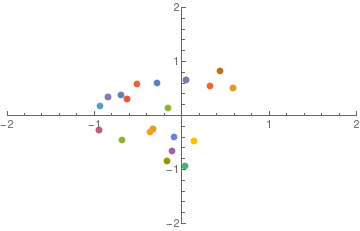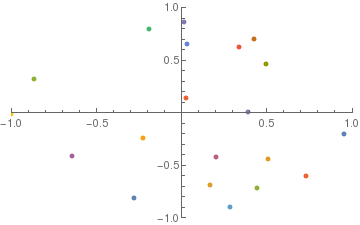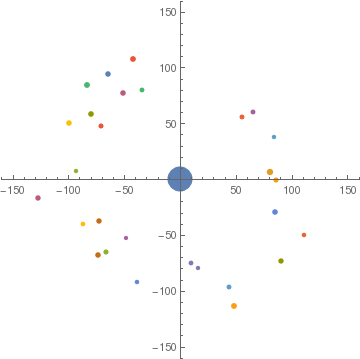# n Body Simulator

## Animations from nBody Simulations

A couple years ago I wrote some Python code for the computational physics course Ph 22, where I simulated $1/r^2$ interactions between n objects using 4th-order Runge-Kutta numerical integration. I didn’t know how to animate with Python back then, so I imported all of the simulated data as csv files into Mathematica, and stitched the gifs together there. Here’s one of the results:After completing the assigned task I decided to add a really simplistic “collision” mechanism, whereby objects would merge (conserving momentum, of course) if their centers were separated by less than the sum of their radii. Here are a couple of animations where the masses were allowed to merge: